Students can Download Maths Chapter 5 Statistics Ex 5.1 Questions and Answers, Notes Pdf, Samacheer Kalvi 7th Maths Book Solutions Guide Pdf helps you to revise the complete Tamilnadu State Board New Syllabus and score more marks in your examinations.

## Tamilnadu Samacheer Kalvi 7th Maths Solutions Term 3 Chapter 5 Statistics Ex 5.1

Question 1.
Fill in the blanks.

1. The mean of first ten natural numbers is ________
2. If the average selling price of 15 books is ₹ 235, then the total selling price is ________
3. The average of the marks 2, 9, 5, 4, 4, 8, 10 is ________
4. The average of integers between -10 to 10 is ________

1. 5.5
2. 3,525
3. 6
4. 0Question 2.
Ages of 15 students in 8th standard is 13, 12, 13, 14, 12, 13, 13, 14, 12, 13, 13, 14, 13, 12, 14.
Find the mean age of the students.
Solution: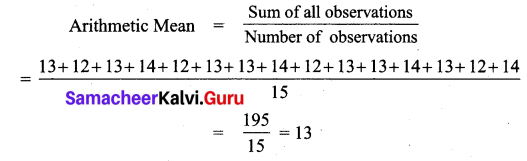= $$\frac { 195 }{ 15 }$$ = 13
Mean age of the students = 13

Question 3.
The marks of 14 students in a science test out of 50 are given below. 34, 23, 10, 45, 44, 47, 35, 37, 41, 30, 28, 32, 45, 39 Find
(i) the mean mark.
(ii) the maximum mark obtained.
(iii) the minimum mark obtained.
Solution: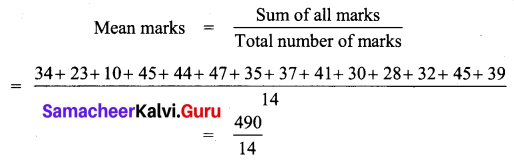Mean marks = 35
(ii) Maximum mark obtained = 47
(iii) Minimum mark obtained = 10Question 4.
The mean height of 11 students in a group is 150 cm. The heights of the students are 154 cm, 145 cm, Y cm, Y + 4 cm, 160 cm, 151 cm, 149 cm, 149 cm, 150 cm, 144 cm and 140 cm. Find the value of Y and the heights of two students.
Solution: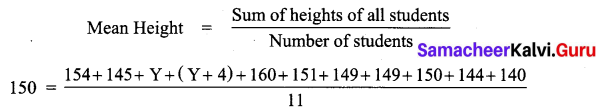150 = $$\frac { 1342+Y+Y+4 }{ 11 }$$
150 = $$\frac { 1346+2Y }{ 11 }$$
150 × 11 = 1346 + 2Y
1650 = 1346 + 2Y
2Y = 1650 – 1346 = 304
Y = $$\frac { 304 }{ 2 }$$ = 152
Height of two students are Y and Y + 4
⇒ 152 and 152 + 4
⇒ 152 cm and 156 cmQuestion 5.
The mean of runs scored by a cricket team in the last 10 innings is 276. If the scores are 235, 400, 351, x, 100, 315, 410,165, 260, 284, then find the runs scored in the fourth innings.
Solution:
Let the runs scored in the fourth innings be x.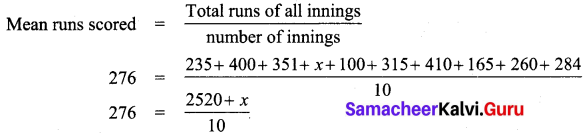276 × 10 = 2520 + x
2760 = 2520 + x
x = 2760 – 2520 = 240
∴ Number of runs scored in the fourth innings = 240

Question 6.
Find the mean of the following data. 5.1, 4.8, 4.3, 4.5, 5.1, 4.7, 4.5, 5.2, 5.4, 5.8, 4.3, 5.6,
Solution: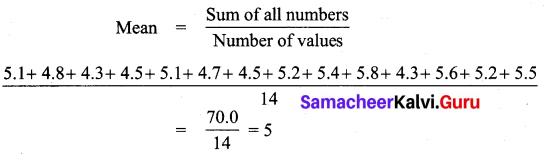Mean = 5

Question 7.
Arithmetic mean of 10 observations was found to be 22. If one more observation 44 was to be added to the data, what would be the new mean?
Solution:
Arithmetic mean of 10 observation is 22.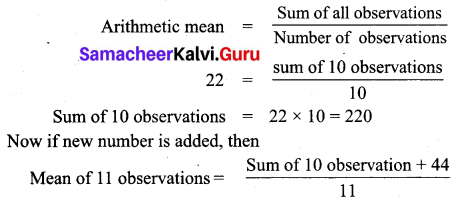= $$\frac { 220+44 }{ 11 }$$ = $$\frac { 264 }{ 11 }$$ = 24
New mean = 24Objective Type Questions

Question 1.
________ is a representative value of the entire data.
(i) Mean
(ii) range
(iii) minimum value
(iv) maximum value
(i) Mean

Question 2.
The mean of first fifteen even numbers is ________
(i) 4
(ii) 16
(iii) 5
(iv) 10
(ii) 16
Hint: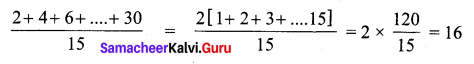Question 3.
The average of two numbers are 20. One number is 24, another number is ________
(i) 16
(ii) 26
(iii) 20
(iv) 40
Ans:
(i) 16
Hint:
$$\frac { x+y }{ 2 }$$ = 20
x + y = 40
24 + y = 40
y = 40 – 24 = 16Question 4.
The mean of the data 12, x, 28 is 18. Find the value of x.
(i) 18
(ii) 16
(iii) 14
(iv) 22
$$\frac { 12+x+28 }{ 3 }$$ = 18